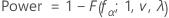# Methods and formulas for Power and Sample Size for Plackett-Burman Design

Select the method or formula of your choice.

## Calculating power

### Calculating power

Minitab assumes that there are no text factors, and therefore no pseudo center points.

Minitab first calculates the degrees of freedom for error (v).

Degrees of freedom for error
ν = (total number of observations – number of parameters estimated)
total number of observations = n * r + c
number of parameters estimated = (number of factors + 1) + 1(if term for center points is included) – (number of main effect terms omitted from the model)
Power### Notation

TermDescription
n number of replicates
r number of runs at the corner points per replicate
cnumber of center points
α significance level
v degrees of freedom for the error
λ noncentrality parameter
fα critical value (upper α point of the F distribution with 1 and ν degrees of freedom)
F(fα ; 1, v, λ)CDF of the F distribution with 1 numerator degree of freedom, v denominator degrees of freedom, and noncentrality parameter λ, evaluated at fα

## Calculating replicates, effects, and center points

If you provide values for power and two other properties, Minitab calculates the unknown fourth property by applying the power equation iteratively. At each iteration, Minitab evaluates the power for the values of the properties that you provide and a trial value of the statistic that you did not provide. Minitab stops when the algorithm reaches the power value that you specified.

When you provide a power value, Minitab may find that no integer number of replicates yields your target power. In such cases, Minitab displays the target value for power alongside the actual power attainable given your specifications. The actual power is a value nearest to, yet greater than, the target power.

## Noncentrality parameter

### Notation

TermDescription
r number of runs at the corner points per replicate
n number of replicates
σ estimated standard deviation
δ effect
λ noncentrality parameter
By using this site you agree to the use of cookies for analytics and personalized content.  Read our policy